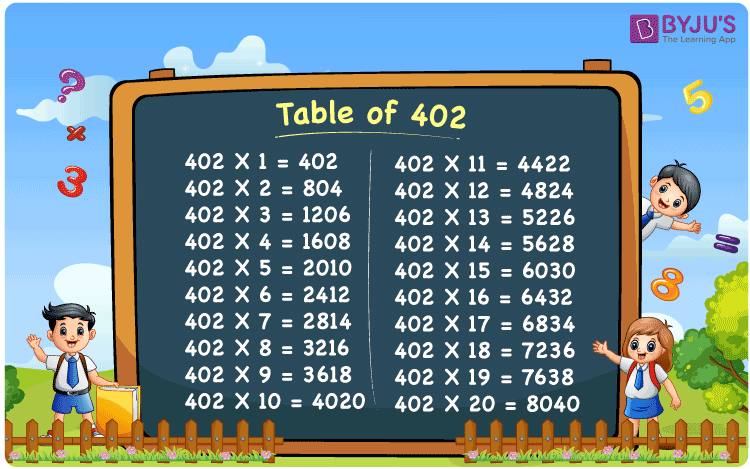Checkout JEE MAINS 2022 Question Paper Analysis : Checkout JEE MAINS 2022 Question Paper Analysis :

# Table of 402

The Table of 402 is given here to help the students quickly check the 402 times table up to 20. The multiplication table of 402 given below is in tabular format, and a PDF is also provided to download. They can download the printable PDF and use the image as a flashcard, which will benefit them to memorise the table of 402.

## Table of 402 Chart

The table chart of 402 is extremely helpful for quickly checking the multiples of 402. The printable image for the table of 402 can be used as a flashcard, assist to do mental maths and solve problems for competitive exams.## What is the 402 Times Table?

The 402 times table is the multiplication of 402 with any natural number. The 402 times table can be obtained both by multiplication and by adding 402 repeatedly. The below chart shows the multiplication table of 402, in both multiplication and addition form.

 402 × 1 = 402 402 402 × 2 = 804 402 + 402 = 804 402 × 3 = 1206 402 + 402 + 402 = 1206 402 × 4 = 1608 402 + 402 + 402 + 402 = 1608 402 × 5 = 2010 402 + 402 + 402 + 402 + 402 = 2010 402 × 6 = 2412 402 + 402 + 402 + 402 + 402 + 402 = 2412 402 × 7 = 2814 402 + 402 + 402 + 402 + 402 + 402 + 402 = 2814 402 × 8 = 3216 402 + 402 + 402 + 402 + 402 + 402 + 402 + 402 = 3216 402 × 9 = 3618 402 + 402 + 402 + 402 + 402 + 402 + 402 + 402 + 402 = 3618 402 × 10 = 4020 402 + 402 + 402 + 402 + 402 + 402 + 402 + 402 + 402 + 402 = 4020

## Multiplication Table of 402

Given below are the first 20 multiples of 402.

 402 × 1 = 402 402 × 2 = 804 402 × 3 = 1206 402 × 4 = 1608 402 × 5 = 2010 402 × 6 = 2412 402 × 7 = 2814 402 × 8 = 3216 402 × 9 = 3618 402 × 10 = 4020 402 × 11 = 4422 402 × 12 = 4824 402 × 13 = 5226 402 × 14 = 5628 402 × 15 = 6030 402 × 16 = 6432 402 × 17 = 6834 402 × 18 = 7236 402 × 19 = 7638 402 × 20 = 8040

## Solved Examples on the Table of 402

Example 1:

Which multiple of 402 is one-third of the sixth multiple of 402?

Solution:

The sixth multiple of 402 = 2412

One-third of 2412 = 2412/3 = 804 = 402 × 2

Hence, the 2nd multiple of 402 is one-third of the sixth multiple.

Example 2:

In a basket, there are 402 apples. How many apples will there be in 15 such baskets?

Solution:

By the given multiplication table of 402

402 × 15 = 3510

Therefore, there are 6030 apples in all the baskets.

## Frequently Asked Questions on the Table of 402

### What is the 402 times table?

The 402 times table is the multiplication table of 402 with given natural numbers. For example, 402 × 1 = 402, 402 × 2 = 804, 402 × 3 = 1206, 402 × 4 = 1608, 402 × 5 = 2010 and so on.

### What times table has 402?

402 comes in the multiplication tables of 1, 2, 3, 6, 67, 134, 201 and 402.

### Does 402 come in 3 times table?

Yes, 3 times 134 is 402.

### Is 402 a prime number?

No, 402 is a composite number. The factors of 402 are 1, 2, 3, 6, 67, 134, 201 and 402.

### What is the prime factorisation of 402?

The prime factorisation of 402 is 2 × 3 × 67.